## Arithmetics is the study of numbers, especially the properties of the traditional operations on them.

Some mathematicians are of the opinion that the doing of mathematics is closer to discovery than invention.

• Bit: The smallest unit in computers. It is either a 1 or a 0.
• Nibble: Half a byte, or 4 bits.
• Byte: 8 bits together form one byte, a number from 0 to 255. Bits in a byte are numbered starting from the right at 0.
• Short: Two bytes put together are 16 bits, forming a number from 0 to 65535. The low byte is the rightmost eight bits.
• Big Endian: Stores data big-end first. When looking at multiple bytes, the first byte (lowest address) is the biggest.
• Little Endian: Stores data little-end first. When looking at multiple bytes, the first byte is smallest.

The number 210, a primorial, is the smallest number divisible by the smallest 4 primes (2, 3, 5, 7) and has 16 divisors (1, 2, 3, 5, 6, 7, 10, 14, 15, 21, 30, 35, 42, 70, 105, 210).

• `a = x - y` where `-` means subtraction, a dyadic use of the symbol
• `a = -y` where `-` means negative, a monadic use of the same symbol

### Peasant Multiplication

In the first column, divide the first number by 2, dropping the remainder if any, until 1 is reached. In the second column, write the numbers obtained by successive multiplication by 2. The answer is found by adding the numbers in the doubling column with odd numbers in their first column.

64 x 61
6461
32122
16244
8488
4976
21952
13904+3904
Result: 3904
61 x 64
6164+64
30128
15256+256
7512+512
31024+1024
12048+2048
Result: 3904

## Binary numbers are a base 2 numeral system.

A binary number is a number expressed in the base-2 numeral system, which uses only two symbols: 0 and 1. Each digit is referred to as a bit. Because of its straightforward implementation in digital electronic circuitry using logic gates, the binary system is used by almost all modern computers and computer-based devices.

### Conversion

Break down the binary value in chunks of 4, multiply each 1 by its equivalent value, either 8, 4, 2 or 1. Add the resulting numbers together to get the result. For example, the value 1100, or (8*1 + 4*1), is equal to C(decimal 12).

`1101 0101(D5)`
11010101Binary
1286432168421Values
128641641Result: 213

### Binary Arithmetic

In the first column, divide the first number by 2 by removing the last bit, until 1 is reached. In the second column, multiply by 2 by adding an extra bit of 0. The answer is found by adding the numbers in the second column with odd numbers in the first column. A binary number ending with 1 is odd.

3519
`100011`` 10011`
` 10001`` 100110`
` 1000`` 1001100`
` 100`` 10011000`
` 10`` 100110000`
` 1``1001100000`
`1010011001`

This example multiplies 35 by 19, to arrive at a result of 665. The result `1010011001` can be deconstucted as:

`10 1001 1001 = 1 + 8 + 16 + 128 + 512 = 665`

#### Multiplication

Binary numbers can be multiplied and divided by multiples of 2, by rotating one bit left to multiply by 2, or one bit right to divide by 2.

 22 44 `ROL` 11 `ROR` 0 0 0 1 0 1 1 0 0 0 1 0 1 1 0 0 0 0 0 0 1 0 1 1

### Logic

AND, or "both", sets individual bits to 0. AND is useful for masking bits, for example, to mask the high order bits of a value AND with \$0F: \$36 AND \$0F = \$06. ORA(OR), or "either one or both", sets individual bits to 1. OR is useful for setting a particular bit, for example, \$80 OR \$08 = \$88. EOR(XOR), or "one or the other but not both", inverts individual bits.

 input output AND ORA EOR 0 1 0 1 1 0 0 0 0 0 1 1 1 1 0 1 0 0 1 1

### Toggling Bits

To activate the 1st, 2nd and 4th bits:

 0 0 0 0 1 0 1 1
```unsigned char num = 0;
num |= (1 << 0);
num |= (1 << 1);
num |= (1 << 3);
```

To deactivate the 1st, 4th and 6th bits:

 1 1 0 1 0 1 1 0
```unsigned char num = 255;
num &= ~(1 << 0);
num &= ~(1 << 3);
num &= ~(1 << 5);
```

`(num >> bit) & 1;`

### Signed Integers

If Bit 7 is not set (as in the first example) the representation of signed and unsigned numbers is the same. However, when Bit 7 is set, the number is always negative. For this reason Bit 7 is sometimes called the sign bit.

 Binary Unsigned Signed 0010 0011 35 35 1010 0011 163 -93 1111 1111 255 -1 1000 0000 128 -128

### Fixed Point

A fixed-point number is a number that has a fixed number of digits after the decimal point. If, for example, we use 8 bits to store a number with decimal points, we could decide to store it this way. The high and low nibbles have the same resolution.

Integer PartDecimal Points
000000000.0
001110103.a
010001014.5

#### Conversion

To turn a regular integer into fixed point, shift left by the number of fractional bits(width << bits), and to turn a fixed point into integer, shift right by the number of fractional bits(width >> bits).

#### Multiplication

To multiply, you do the multiply, and then you shift right by the number of fractional bits.

`(3.8 * 2.0) >> 8`

#### Division

To divide, you first shift the numerator left by the number of fractional bits, then you do the division.

`(3.8 << 8) / 2.0`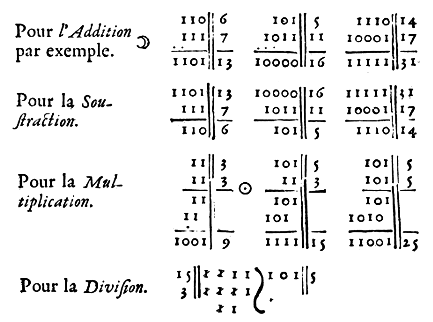## Hexdecimal numbers are a base 16 numeral system.

Hexadecimal numerals are widely used by computer system designers and programmers because they provide a human-friendly representation of binary-coded values. Each hexadecimal digit represents four bits.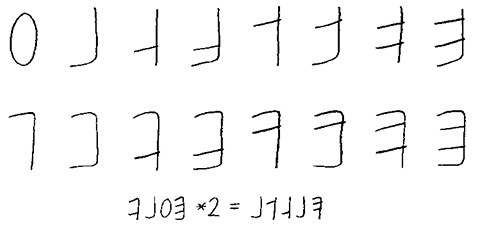### Finger Counting

Finger binary is a system for counting and displaying binary numbers on the fingers of one or more hands. It is possible to count from 0 to 31 using the fingers of a single hand. In the binary number system, each numerical digit has two possible states(0 or 1) and each successive digit represents an increasing power of two.

PinkyRingMiddleIndexThumb
Value124816

For example, the number 10 is expressed by folding the index and ring finger, the number 20 is expressed by folding the thumb and the middle finger.A way to look at this system is to consider the hand as an abacus, where the little finger has a value of 1, the ring finger has a value of 2, the middle finger has a value of 4, and the index has a value of 8. Numbers are made by adding the value of the pointed fingers.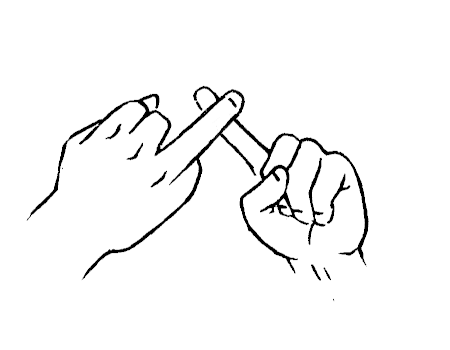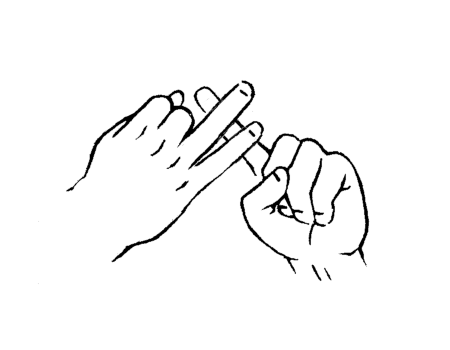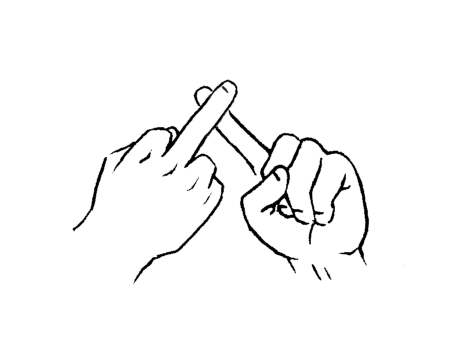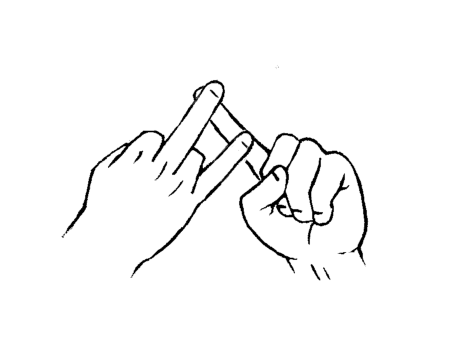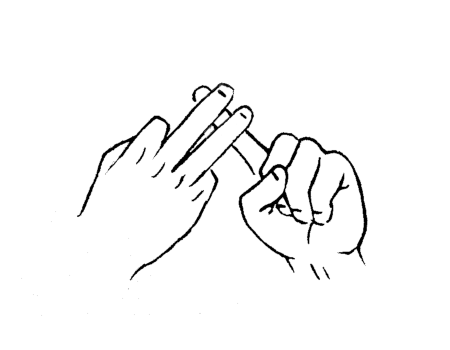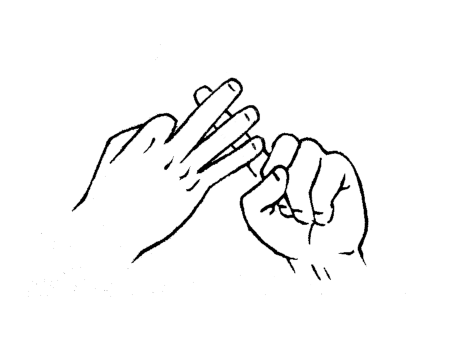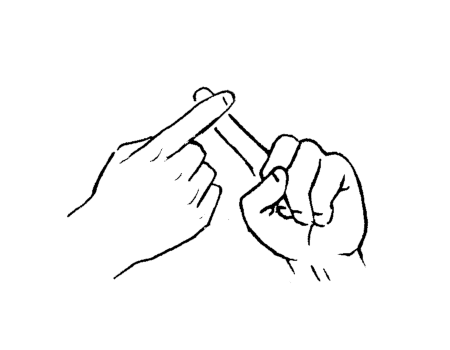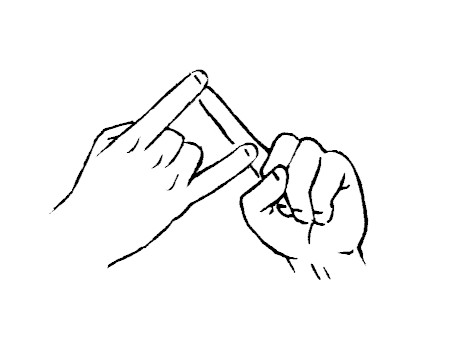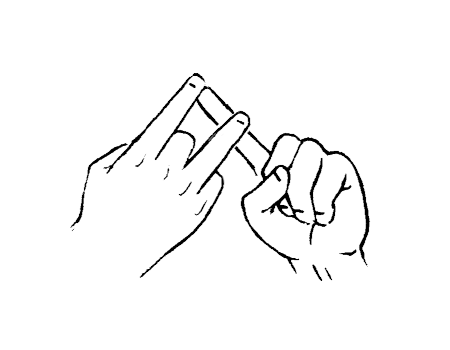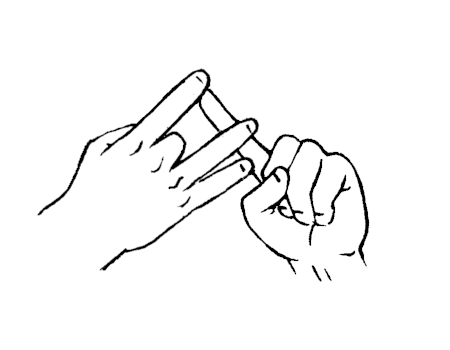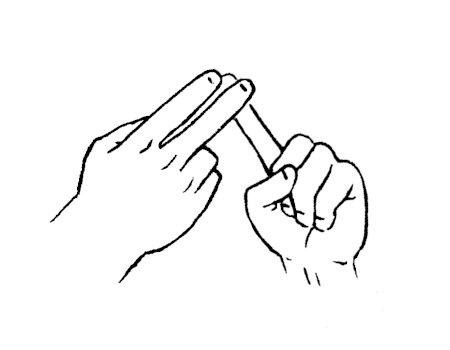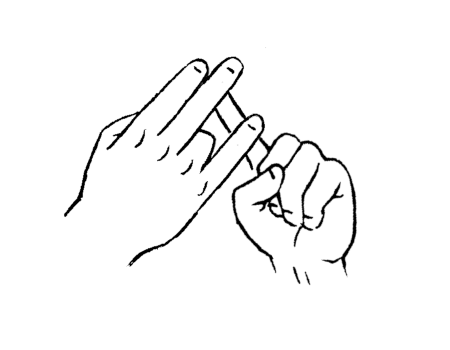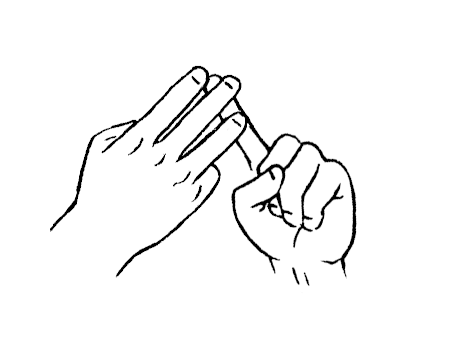To sign numbers, such as the number two, which would be hard to do by sheer positioning of the finger, open your palm, and use your left index finger to push the ring finger out of your right palm. Don't try to extend the ring finger out on its own.

### Verbal Counting

A 1A A0 A00 ha hateen haty handred be beteen bety bendred ce ceteen cety cendred de deteen dety dendred he heteen hety hendred fe feteen fety fendred

You can find a larger table, the midi table and the ascii table.

 0 0000 4 0100 8 1000 C 1100 1 0001 5 0101 9 1001 D 1101 2 0010 6 0110 A 1010 E 1110 3 0011 7 0111 B 1011 F 1111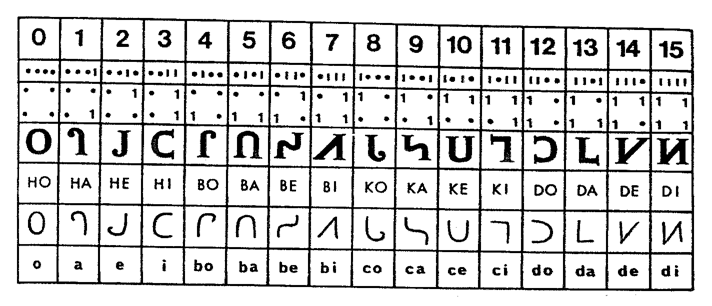## Numbers

Hexadecimal numerals are widely used by computer system designers and programmers because they provide a human-friendly representation of binary-coded values. Uxn gestures differenciate from traditional ASL numbers which are used only in the names of labels.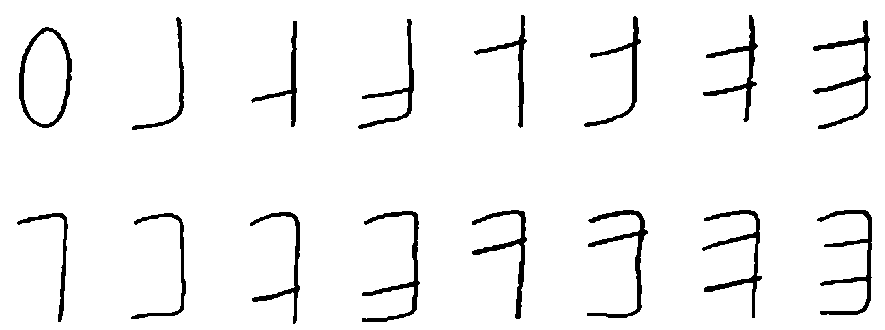## A prime number cannot be divided by any other number, apart from itself and one.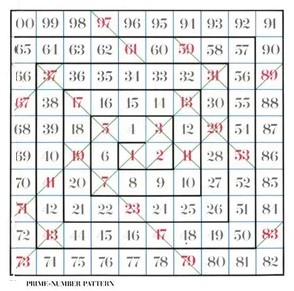To find the prime factorization of a number, start by dividing the number by the first prime number 2 and continue dividing by 2 until you get a decimal or remainder. Then divide by 3, 5, 7, etc. until the only numbers left are prime numbers.

NumberPrimes
235
6110
375013
2250123

Multiplying two numbers is the same as adding the counts of each prime factors, and division is the same as subtracting the counts. For example, using numbers made up of the 3 first primes(2, 3, 5), 2250 is equal to `2^1 x 3^2 x 5^3`.

### Storage

An interesting part of primes is the ability to encode data, values can be encoded as exponents to a number's prime factors, or as values to registers as in Fractran. For example, the letters of the word "HELLO" can be stored as ascii exponents to the first five primes:

 H E L L O 2^72 3^69 5^76 7^76 11^79

The resulting number storing the values of each character of "HELLO":

```1639531486723067852359816964623169016543137549
4122401687192804219102815235735638642399170444
5066082282398711507312101674742952521828622795
1778467808618104090241918575825850806280956250
0000000000000000000000000000000000000000000000
0000000000000000000000000
```

Extra letters can be appended by multiplying with a number reducible with the following prime, for example `* 13^33` would make our message "HELLO!". Letters can also be removed by dividing by one of its factors, for example `/ 3^69` would make our message "HLLO!".

### Uxntal Routine

To find if a number is prime in Uxntal:

```@is-prime ( value* -- bool )

DUP2 #0002 EQU2 ,&pass-end JCN
DUP #01 AND #00 EQU ,&fail-end JCN
#0003
&loop
OVR2 OVR2 DUP2 MUL2 LTH2 ,&pass JCN
OVR2 OVR2 DIV2k MUL2 EQU2 ,&fail JCN
INC2 INC2 ,&loop JMP
&fail POP2 &fail-end POP2 #00 JMP2r
&pass POP2 &pass-end POP2 #01 JMP2r
```

## A fraction represents a part of a whole.

A fraction consists of a numerator displayed above a line, and a denominator below.

NumberPrimes
235
2/3110
27/25032
100/9222

Multiplying a number by a fraction, is the same as adding the prime numerators and subtracting the prime denominators. For example, multiplying 18, which is made of `2^1 x 3^2`, by `2/3` means incrementing prime 2, and decrementing the prime 3, or `2^2 x 3^1`.

• An proper fraction must be less than 1, like `3/4` and `7/12`.
• An improper fraction is more than 1, like `9/2` and `13/4`.
• The reciprocal of a fraction is another fraction with the numerator and denominator exchanged, like `3/7` for `7/3`.

To experiment with primes, have a look at Fractran.

### Reducing

Dividing the numerator and denominator of a fraction by the same non-zero number yields an equivalent fraction: if the numerator and the denominator of a fraction are both divisible by a number (called a factor) greater than 1, then the fraction can be reduced to an equivalent fraction with a smaller numerator and a smaller denominator.

#### Recursive Method

```function gcd(a, b)
if b = 0
return a
else
return gcd(b, a mod b)```

## Comparing

Comparing fractions with the same positive denominator yields the same result as comparing the numerators.

To add fractions containing unlike quantities , it is necessary to convert all amounts to like quantities.

```1/4 + 1/3
1*3/4*3 + 1*4/3*4
3/12 + 4/12 = 7/12```

The process for subtracting fractions is, in essence, the same as that of adding them: find a common denominator, and change each fraction to an equivalent fraction with the chosen common denominator.

## Multiplication

To multiply fractions, multiply the numerators and multiply the denominators.

`2/3 * 3/4 = 6/12`

## In Postfix Notation, the operators follow their operands.

In Postfix calculators, no equals key is required to force computation to occur. To learn more about a programming language using Postfix at its core, see Forth.

prefix notationinfix notationpostfix notation
`+ 1 * 2 3+ 1 67` `(1 + (2 * 3)(1 + 6 ) 7` `1 2 3 * +1 6 + 7`

For instance, one would write `3 4 +` rather than `3 + 4`. If there are multiple operations, operators are given immediately after their second operands. The expression written `(5 + 10) * 3` in conventional notation would be written `10 5 + 3 *` in reverse Polish notation.

 3 10 5 + * operation stack 3 10 5 15 45 3 10 3 3

The automatic stack permits the automatic storage of intermediate results for use later: this key feature is what permits Postfix calculators to easily evaluate expressions of arbitrary complexity: they do not have limits on the complexity of expression they can evaluate.

Brackets and parentheses are unnecessary: the user merely performs calculations in the order that is required, letting the automatic stack store intermediate results on the fly for later use. Likewise, there is no requirement for the precedence rules required in infix notation.

Incoming: firth logic language